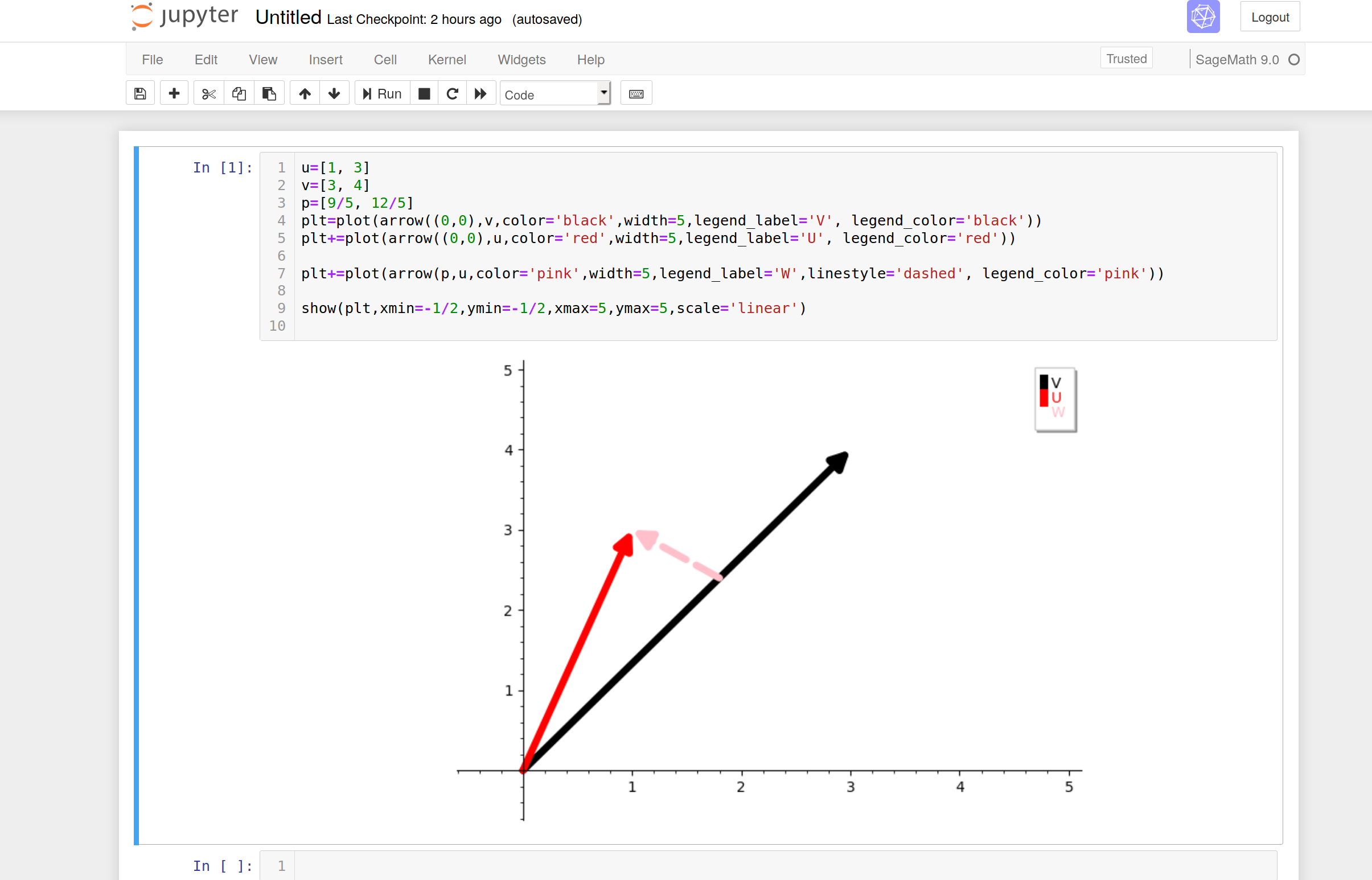# Centering the graphics output

Is there a way to ask Sagemath to center the graphics in the page ?

edit retag close merge delete

Sort by » oldest newest most voted

Hi Cyrille

What OS and What Sagemath notebook ?

look here

for me with Ubuntu 18.04 and Sagemath notebook 9.0 it is working with putting:

.output_png {
display: table-cell;
text-align: center;
margin:auto;
}
.prompt
display:none;
}


at the end in my css file:./local/lib/python3.7/site-packages/notebook/static/custom/custom.css

like it is recommended in the link above.more

I also have a question that I allow myself to add in comment in your question(because it is in relation): how to do so that in the code following the x-axis scale is the same as the y-axis? (so that the angle between the pink vector and the black vector appears at right angles)

u=[1, 3]
v=[3, 4]
p=[9/5, 12/5]
plt=plot(arrow((0,0),v,color='black',width=5,legend_label='V', legend_color='black'))
plt+=plot(arrow((0,0),u,color='red',width=5,legend_label='U', legend_color='red'))

plt+=plot(arrow(p,u,color='pink',width=5,legend_label='W',linestyle='dashed', legend_color='pink'))

show(plt,xmin=-1/2,ymin=-1/2,xmax=5,ymax=5,scale='linear')

1

I would add aspect_ratio=1 as an option in show.

Thank you Juanjo, I got a nice right angle now with the option you recomend ;-)